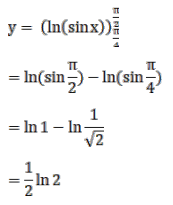# Mark against the correct answer in the following:

Question:

Mark $(\sqrt{)}$ ) against the correct answer in the following:

$\int_{\pi / 4}^{\pi / 2} \cot x d x=?$

A. $\log 2$

B. $2 \log 2$

C. $\frac{1}{2} \log 2$

D. none of these

Solution: